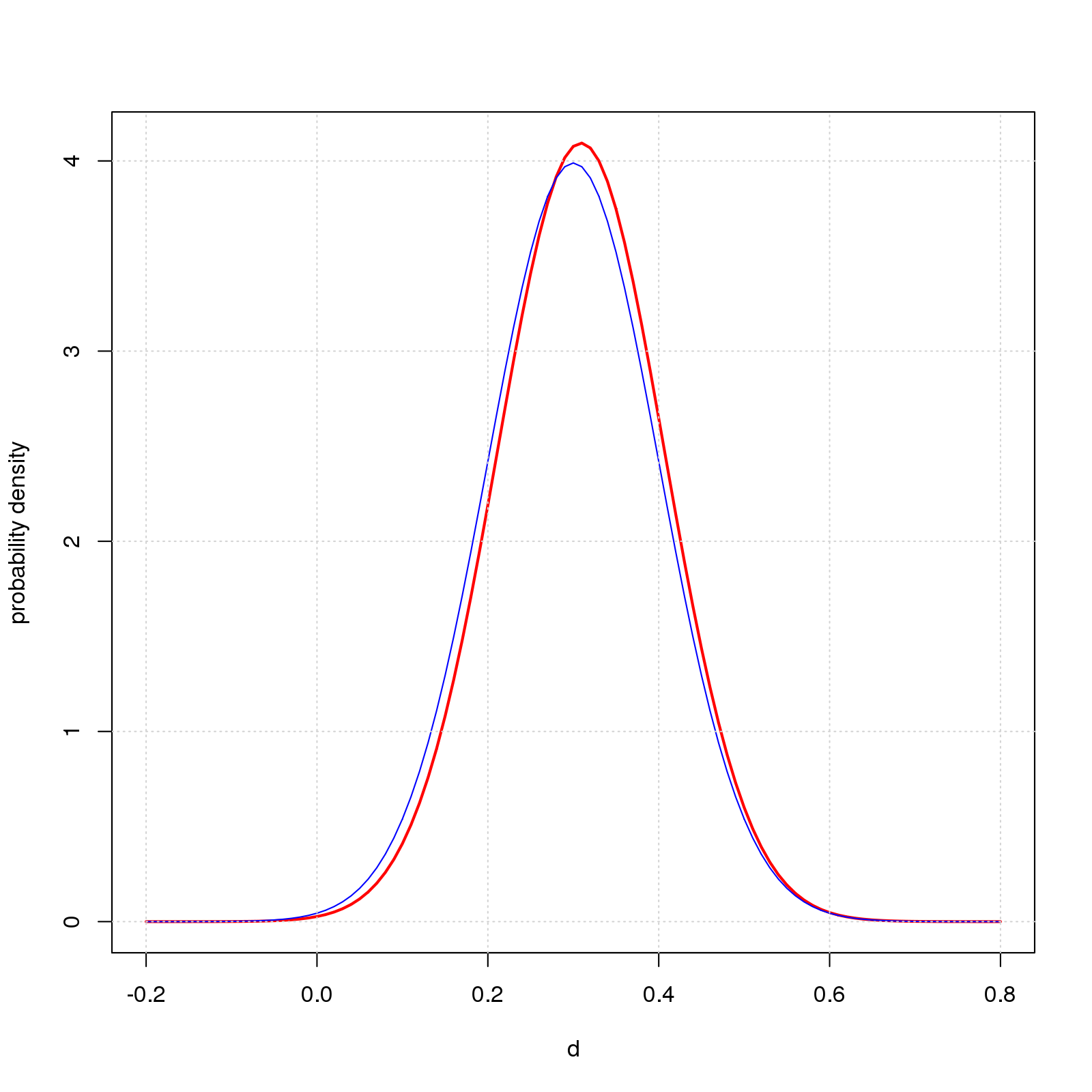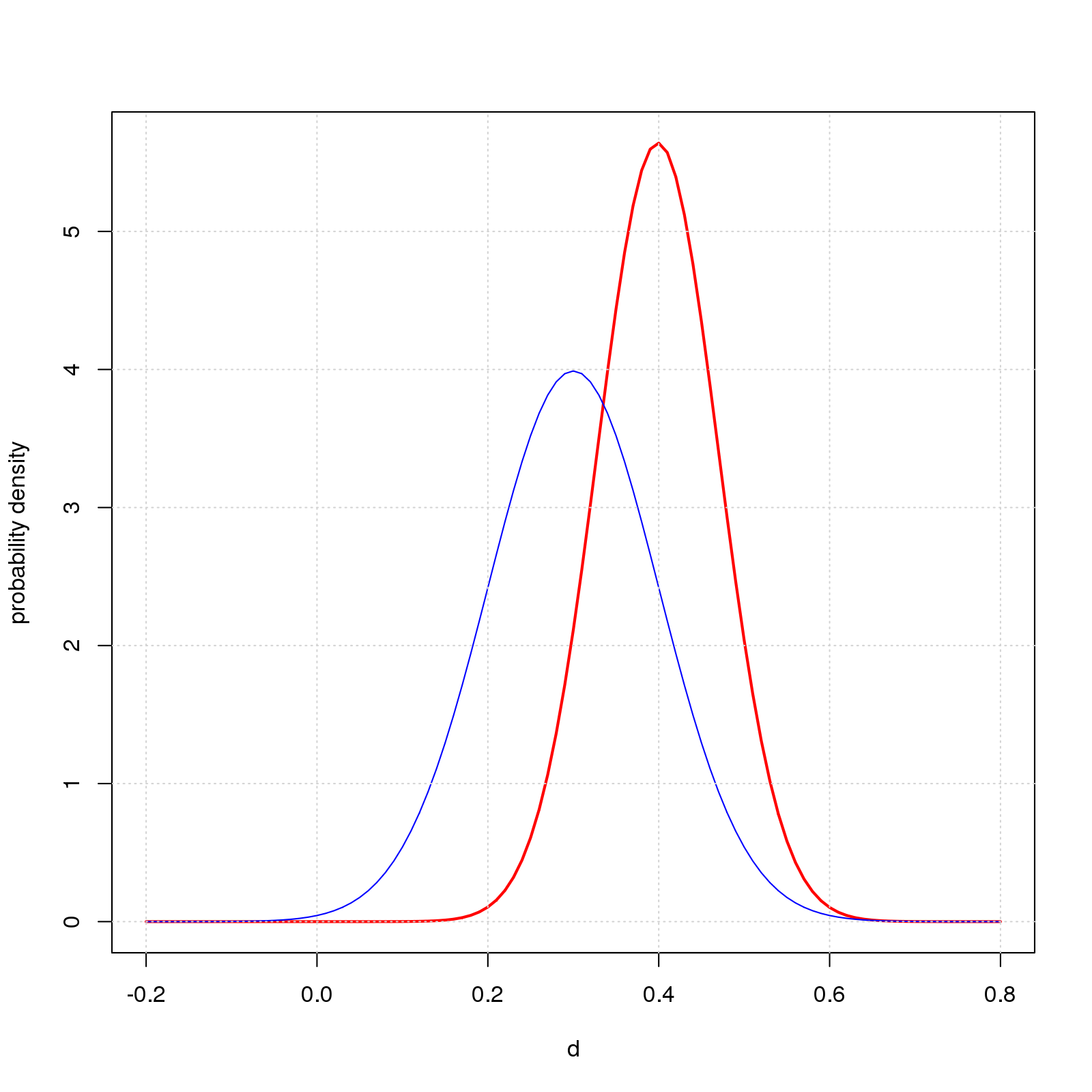This working paper shows an R implementation of Bayes’s formula as used in the blog post What’s the True Effect Size? It Depends What You Think to estimate true effect size in two group difference-of-mean studies. The main point is that the implementation is really, really simple. With all the fuss and bother about Bayesian methods, I imagined it would be incredibly hard to code. Happily not.

## Bayes’s Formula

The line of math below is Bayes’s formula for the example at hand. An important nuance is that the “probabilities” are probability densities - think R’s dnorm.

$P(d_{pop} \mid d_{obs}) = \frac {P(d_{pop}) \times P(d_{obs} \mid d_{pop}) } {P(d_{obs})}$

1. The term on the left hand side is what we’re trying to compute, namely, the posterior probability distribution of $$d_{pop}$$ for a given value of $$d_{obs}$$ ($$0.5$$ in the blog post’s running example).

2. The first term on the right hand side, $$P(d_{pop})$$, is the prior. For the normal prior in Figures 1 and 2 of the post, this is R’s dnorm with $$mean=0.3$$ and $$sd=0.1$$.

3. The next term, $$P(d_{obs} \mid d_{pop})$$, is the probability distribution of $$d_{obs}$$ for a given $$d_{pop}$$. For the two group difference-of-mean studies in the post, this is a noncentral t-distribution centered on $$d_{obs}$$.

4. The denominator, $$P(d_{obs})$$, is the probability of a given value of $$d_{obs}$$ across all values of $$d_{pop}$$. This is the integral from $$-\infty$$ to $$\infty$$ of the numerator in Bayes’s formula.

## R Implementation

Below is R code to compute the posterior for the examples in this post. The code works: just copy-and-paste into an R session and it’ll plot two graphs similar to Figures 1 and 2 in the blog post. The code is also available in the file R/baysx.R in my GitHub repo.

## Return function for posterior probability of d.pop given d.obs for two group
## difference-of-mean studies with equal sample size and unit standard deviation
##   n is sample size per group
##   d.obs is standardized observed effect size
##   prior is function of d.pop, eg, function(d.pop) dnorm(d.pop,mean=0.3,sd=0.1)
##
posterior=function(n,d.obs,prior) {
## probability of d.obs given d.pop for examples at hand. dd2t defined below
P_obsGIVENpop=function(d.pop) dd2t(n,d.pop,d.obs);
## numerator in Bayes formula
numerator=function(d.pop) prior(d.pop)*P_obsGIVENpop(d.pop);
## denominator in Bayes formula
P_obs=integrate(function(d.pop) numerator(d.pop),-Inf,Inf)\$value;
P_popGIVENobs=function(d.pop) numerator(d.pop)/P_obs;
}

## probability density of noncentral t in terms of n, d.pop, d.obs
dd2t=function(n,d.pop,d.obs) {
df=2*(n-1);
t=d.pop*sqrt(n/2);
sqrt(n/2)*suppressWarnings(dt(t,df=df,ncp=sqrt(n/2)*d.obs));
}

## examples with normal prior as in Figures 1 and 2 of the post
##   each graph plots the posterior for the given value of n,
##   as well as the prior (dnorm(d.pop,mean=0.3,sd=0.1)) for reference
## n=10
dev.new();
dpost=posterior(n=10,d.obs=0.5,prior=function(d.pop) dnorm(d.pop,mean=0.3,sd=0.1));
curve(dpost,from=-0.2,to=0.8,col='red',lwd=2,xlab='d',ylab='probability density');
grid();
## n=200;
dev.new();
dpost=posterior(n=200,d.obs=0.5,prior=function(d.pop) dnorm(d.pop,mean=0.3,sd=0.1));
curve(dpost,from=-0.2,to=0.8,col='red',lwd=2,xlab='d',ylab='probability density');
grid();

The resulting graphs areThe code exploits an advanced R feature that may be unfamiliar to some readers, namely, the ability to treat functions like other kinds of data. You can store a function in a variable, pass a function as an argument to another function, create a new function on-the-fly and store it in a variable, call the newly created function via the variable, and return a function as the result of a function.

I use this feature repeatedly.

1. I pass the prior probability function (dnorm(d.pop,mean=0.3,sd=0.1)) to posterior as the argument prior.

2. Each line of posterior creates a function: e.g., the line P_obsGIVENpop=function(d.pop) dd2t(n,d.pop,d.obs); creates a function of d.pop that calls the function dd2t on variables n, d.pop, and d.obs. A further detail is that variables n and d.obs are bound to the values passed into posterior as arguments, while d.pop is free and gets its value later when the new function P_obsGIVENpop is called.

3. The final result is likewise a function of d.pop: function(d.pop) numerator(d.pop)/P_obs;

4. The examples store the function in the variable dpost, then use curve (a base R function) to call dpost and plot the result.

## Web Resources I Found Helpful

The web is awash in Bayesian material, but I found most of it inscrutable. Here are web pages that were simple enough to get me started on the path to understanding. Thanks! I appreciate the helping hand!